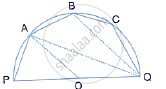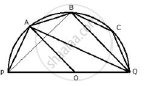Share

# The Given Figure Shows a Semi-circle with Centre O and Diameter Pq. If Pa = Ab and ∠Bcq = 140°; Find Measures of Angles Pab and Aqb. Also, Show that Ao is Parallel to Bq. - Mathematics

Course

#### Question

The given figure shows a semi-circle with centre O and diameter PQ. If PA = AB and ∠BCQ =140°; Find measures of angles PAB and AQB. Also, show that AO is parallel to BQ.#### SolutionJoin PB.
∠BPQ + ∠BCQ = 180°

⇒  ∠BPQ + 140°  =  180°
⇒ ∠BPQ = 40°  ……. (1)
Now in ΔPBQ,
∠PBQ  + BPQ + ∠BQP = 180°
⇒ 90° + 40° + ∠BQP = 180°
⇒  ∠BQP = 50°
∠PQB + ∠PAB = 180°
⇒ 50° + ∠PAB =  180°
⇒ ∠PAB = 130°

ii) Now in  ΔPAB,
∠PAB + ∠APB  + ABP = 180°
⇒ 130° + ∠APB  + ∠ABP = 180°
⇒  ∠APB + ∠ABP = 50°
But
∠APB = ∠ABP ( ∵ PA = PB)
∴  ∠APB = ∠ABP = 25°
∠BAQ = ∠BPQ = 40°
∠APB = 25°  = ∠AQB  (angles in the same segment)
∴  ∠AQB = 25° ………. (2)

iii) Arc AQ subtends  ∠AOQat the centre and ∠APQ at the remaining part of the circle.
We have,
∠APQ =  ∠APB + ∠BPQ ……. (3)
From (1), (2) and (3), we have
∠APQ = 25° + 40° = 65°
∴ ∠AOQ =  2∠APQ  = 2 × 65° = 130°
Now in ΔAOQ,
∠OAQ =  ∠OQA = ( ∵ OA = OQ)
But
∠ OAQ  + ∠OQA + ∠AOQ  = 180°
⇒ ∠OAQ  + ∠OAQ  +130° = 180°
⇒ 2 ∠OAQ  = 50°
⇒  ∠OAQ = 25°
∴ ∠OAQ  =  ∠AQB
But these are alternate angles.
Hence, AO is parallel to BQ.

Is there an error in this question or solution?